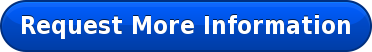In my fifth grade math class, students learned about fractions as they created works of art. When I first introduced the unit on fractions, I noticed my students were struggling with the concept. In an effort to combat the initial nerves of learning something new and complex, I asked students to use a ruler. I discovered that the students first had to learn to properly use the ruler itself, to understand about increments of an inch. Then, they began drawing straight lines on an x/y axis. The final result was a beautiful piece of art made one straight line at a time! Here’s how it worked:

Students first learned the sixteenths on the ruler, then reduced that to learn where each eighth and quarter is. After drawing an x/y axis, students marked every eighth inch on each axis. Then students connected the farthest point on the x axis to the point nearest to the center on the Y axis. The students continued down connecting points along the way. They completed this task with each quadrant. The students were amazed to find that using their ruler to draw straight lines resulted in a final product of curved lines.

This project helped the class better understand the unit because students learned how to split a space evenly to create fractions. It also taught them how to add fractions. One student told me, "I thought this was very interesting because we did the drawings and then we learned during the drawings and we didn't even notice." Another added, "I think that the line drawings are much better than worksheets because it's fun, creative, and teaches measurement."

This project was useful when the students began estimating sums of fractions to the nearest half inch because they could visualize the ruler and understand where the fractions would fall on the ruler. These students said it best,  "I like how you are doing a challenging learning experience but also having so much fun!" and "I think when you learn something through a fun activity, you learn it better." I agree!Topics: Education, learning, math, art